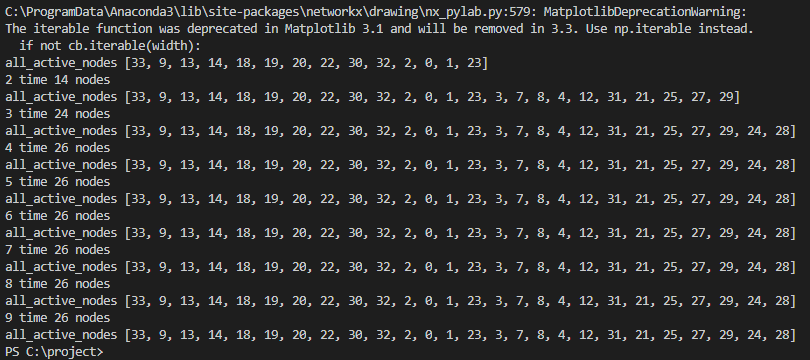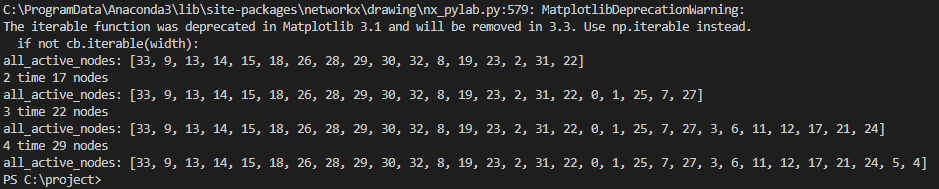• SI模型，影响力传播模型，传染病模型，信息传播模型python代码 SI模型，影响力传播模型，传染病模型，信息传播模型python代码 SI模型，影响力传播模型，传染病模型，信息传播模型python代码 SI模型，影响力传播模型...
• ## SIR信息传播模型

千次阅读 热门讨论 2020-06-12 17:22:41
SIR信息传播模型SIR模型及python复现SIR模型SIR数学模型 SIR模型及python复现 SIR模型 SIR模型是传染病模型中的经典模型，可以用在传染病过程中的模拟预测，也可以用作抽象表达社交网络中的信息传播过程，本文讲述的...


SIR信息传播模型
SIR模型及python复现SIR模型SIR数学模型传播动力学方程python实现

模拟社交网络中SIR模型的信息传播过程

SIR模型及python复现
SIR模型
SIR模型是传染病模型中的经典模型，可以用在传染病过程中的模拟预测，也可以用作抽象表达社交网络中的信息传播过程，本文讲述的是后者。 SIR模型将社交网络中的节点分为三类：

S

S

类，易感染者，指未接收信息且不具备传播能力的节点；

I

I

类，感染者，指接收信息且具备传播能力的节点；

R

R

类，免疫者，指接收信息后退出传播过程的节点。 SIR模型的传播机制如下图所示：其中，

β

β

代表感染率，即时刻

t

t

时单位时间内

I

I

类节点新增的感染个体与

S

S

类节点个数成比例，比例系数为

β

β

；

γ

γ

代表免疫率(恢复率)，即时刻

t

t

时单位时间内

R

R

类节点新增的免疫个体与

R

R

类节点个数成比例，比例系数为

γ

γ

。
SIR数学模型
传播动力学方程
我们将上述传播模型表达为动力学方程。这里设置节点总数为

N

N

，其他三类节点初始数目分别为

S

、

I

、

R

S、I、R

且

N

=

S

+

I

+

R

N=S+I+R

。则有下列方程

{

d

S

d

t

=

−

β

S

I

N

,

d

I

d

t

=

β

S

I

N

−

γ

I

,

d

R

d

t

=

γ

I

其中，

I

N

\dfrac{I}{N}

代表

S

S

类与

I

I

类的接触率。
python实现
from scipy.integrate import odeint
import numpy as np
import matplotlib.pyplot as plt

def sir_model(input_v, t):
s, i, r = input_v
n = s + i + r
ds_dt = - beta * s * (i / n)
di_dt = beta * s * (i / n) - gamma * i
dr_dt = gamma * i
return [ds_dt, di_dt, dr_dt]

if __name__ == '__main__':
# 模型输入值
N = 10000  # 设定总节点数量
S0 = 9980  # S类节点数量
I0 = 18  # I类节点数量
R0 = N - S0 - I0  # R类节点数量
input_value = (S0, I0, R0)
beta = 0.5  # 设定感染率
gamma = 0.1  # 设定免疫率
# 设定步长 即传播次数
times = np.linspace(0, 60, 60)
# 求解微分方程
result = odeint(sir_model, input_value, t=times)
# 画图
plt.plot(result[:, 0], '-ro', label='S')
plt.plot(result[:, 1], '-b^', label='I')
plt.plot(result[:, 2], '-gs', label='R')
plt.legend(loc=0)
plt.xlabel('times')
plt.ylabel('number')
plt.show()

实现结果如下图所示。模拟社交网络中SIR模型的信息传播过程
在这里由于没有数据集，所以只能用python中的Networkx库，这里我们使用BA无标度网络模型(个人认为比较接近于现实社交网络)。
import networkx as nx
import numpy as np
import pandas as pd
import matplotlib.pyplot as plt
import matplotlib.animation as animation
import random
# 确保 中文 和 -
plt.rcParams['font.sans-serif'] = ['SimHei']
plt.rcParams['axes.unicode_minus'] = False

def update_node_status(G, node, beta, gamma):
"""
更改节点状态
:param G: 输入图
:param node: 节点序数
:param beta: 感染率
:param gamma: 免疫率
"""
# 如果当前节点状态为 感染者(I) 有概率gamma变为 免疫者(R)
if G.nodes[node]['status'] == 'I':
p = random.random()
if p < gamma:
G.nodes[node]['status'] = 'R'
# 如果当前节点状态为 易感染者(S) 有概率beta变为 感染者(I)
if G.nodes[node]['status'] == 'S':
p = random.random()
if p < beta:
G.nodes[node]['status'] = 'I'
break

def update_network_data(G, beta, gamma):
"""
更改图数据
:param G: 输入图
:param beta: 感染率
:param gamma: 免疫率
"""
for node in G:
update_node_status(G, node, beta, gamma)

def initial_network_data(G, i_num, r_num):
"""
初始化图数据
:param G: 输入图
:param i_num: 感染者数量
:param r_num: 免疫者数量
"""
# 感染节点集
i_set = set(random.sample(G.nodes, i_num))
# 免疫节点集
r_set = set(random.sample(G.nodes, r_num))
# 两个集合不能重复
while r_set & i_set:
r_set = set(random.sample(G.nodes, r_num))
# 初始化节点状态
for node in G:
if node in i_set:
G.nodes[node]['status'] = 'I'
elif node in r_set:
G.nodes[node]['status'] = 'R'
else:
G.nodes[node]['status'] = 'S'

def count_node(G):
"""
计算当前图内各个节点的数目
:param G: 输入图
:return: 各个节点数目
"""
s_num, i_num, r_num = 0, 0, 0
for node in G:
if G.nodes[node]['status'] == 'S':
s_num += 1
elif G.nodes[node]['status'] == 'I':
i_num += 1
else:
r_num += 1
return s_num, i_num, r_num

def draw_network(G):
"""
输出初始网络节点分布
:param G: 输入图
"""
# 设置图大小
fig, ax = plt.subplots(figsize=(12, 8))
ax.set_title("易感染者-感染者-免疫者节点初始分布")
pos = nx.spring_layout(G, scale=1)
nx.draw(G, pos=pos, with_labels=True, font_color='white', edge_color='grey',
node_color=[color_dict[ba.nodes[node]['status']] for node in G])

def draw_node_trend(G, beta, gamma):
"""
输出各类节点趋势
:param G: 输入图
:param beta: 感染率
:param gamma: 免疫率
"""
# 设定传播步长
t_list = np.linspace(1, 24, 24)
# 开始模拟传播
for t in range(len(t_list)):
# 计算并存储当前各个节点数目
node_list.append(count_node(G))
update_network_data(G, beta, gamma)
# 整理数据
df = pd.DataFrame(data=node_list, index=t_list, columns=['S', 'I', 'R'])
# 显示数据曲线
df.plot(figsize=(8, 6), color=[color_dict.get(x) for x in df.columns])
plt.ylabel('nodes(节点数)')
plt.xlabel('days(天数)')
plt.title('易感染者-感染者-免疫者节点趋势')
plt.savefig('SIR_model')
plt.show()

def update_graph(i, G, ax, pos, beta, gamma):
"""
动态更新节点
:param i: 输入帧
:param ax: 输入图参数
:param G: 输入图
:param beta: 感染率
:param gamma: 免疫率
"""
i = int(i)
ax.set_title("第" + str(i + 1) + "天 易感染者-感染者-免疫者节点分布")
ax.axis('off')
plt.box(False)
if i == 1:
# 第一天  初始节点分布  直接画出
nx.draw(G, with_labels=True, font_color='white', edge_color='grey',
node_color=[color_dict[ba.nodes[node]['status']] for node in G], pos=pos)
else:
# 以后变化 需要演变节点
update_network_data(G, beta, gamma)
nx.draw_networkx_nodes(G, with_labels=True, font_color='white', edge_color='grey',
node_color=[color_dict[ba.nodes[node]['status']] for node in G], pos=pos)

def draw_network_trend(G, beta, gamma, days):
"""
输出网络动态变化视频
:param G: 输入图
:param beta: 感染率
:param gamma: 免疫率
:param days: 需要的时间(迭代次数)
"""
fig, ax = plt.subplots(figsize=(12, 8))
pos = nx.spring_layout(G, scale=1)
ani = animation.FuncAnimation(fig, update_graph, frames=days,
fargs=(G, ax, pos, beta, gamma), interval=300)
writer = animation.FFMpegWriter()
ani.save('network_trend.mp4', writer=writer)

if __name__ == '__main__':
# 总人数
N = 10000
# 易感染者人数
s = 9980
# 感染者人数
i = 18
# 免疫者人数
r = N - s - i
# 各个节点数目列表
node_list = []
# 节点颜色
color_dict = {'S': 'blue', 'I': 'red', 'R': 'green'}
# 创建BA无标度网络
ba = nx.barabasi_albert_graph(N, 3, seed=1)
# 初始化网络节点
initial_network_data(ba, i, r)
# 输出节点趋势图
draw_node_trend(ba, 0.4, 0.1)
# 初始节点分布图
# 输出初始节点网络图
# draw_network(ba)
# 输出网络动态变化图
# draw_network_trend(ba, 0.2, 0.15, 50)

上述代码得到的结果如下图所示。这里说一下，如果有数据集可以修改一下这个代码，将数据集中的边集和节点集输入Networkx中的空白图中，剩余可以不改变，也可以模拟SIR模型在真实社交网络下的传播过程。 从SIR模型中我们可以看到，

β

β

和

γ

γ

在很大程度会影响信息传播过程。如果利用真实数据集，也要注意不同的

β

β

和

γ

γ

值，会有不同的结果。同时，利用好真实数据集，我们可以求出近似真实值

β

β

和

γ

γ

值。 另外在上述主程序代码的最后几行，我增加了生成网络初始分布图和动态实现社交网络中节点变化的函数。动态实现那部分需要一个ffmpeg的软件才可以生成。
展开全文python
• 信息传播模型能够揭示信息传播的规律，从而达到对传播过程进行预测的目的，因此社交网络信息传播模型的研究具有重要意义。针对经典传染病模型的不足进行了分析，结合社交网络的网络拓扑特点，考虑了网络中用户的不同...社交网络
• 本文以Device-to-Device移动社交网络为研究对象,首先分析该网络中用户参与信息传播的特点,然后在传染病模型的基础上构建适合此网络特点的信息传播模型,并利用该模型分析真实Device-to-Device环境中的信息传播过程。...
• 提出了一种新的无拓扑结构的社交网信息传播模型，简称 NT-II，并使用表达学习方式，构建了 2 个隐藏的空间：用户影响空间和用户兴趣空间，每个用户和每个传播项都映射成空间中的向量。模型在预测用户接收传播项的...
• 传统的社会网络信息传播模型假设整个网络结构是已知的，并在已知的网络上分析信息的动态传播，然而实际的社会网络往往是不完全的。为了研究信息在不完全社会网络上的信息传播，提出了一种基于核函数的信息传播模型。...社会网络 学习算法 时间戳 核函数
• 由于灰色理论在解决少数据、贫信息、不确定性问题具有一定的优势，本文提出了一种基于灰色神经网络的微博网络信息传播模型．模型将传播过程中动态、不确定因素作为扩展BP神经网络的输入参数，进行训练并预测信息传播...
• 摘要：主要讲解一些基本的信息传播模型，以及IC模型、SI模型和SIR模型的python实现及可视化。 author：xiao黄 缓慢而坚定的生长 最后更新于2020.10.06
摘要：主要讲解一些基本的信息传播模型，以及IC模型、SI模型和SIR模型的python实现及可视化。

2021.10.06更新有需要的可以点击传送门

2020.09.26更新更新了SIR模型的实现，请点击传送门，就不放在这篇博客里了

2020.09.03更新更新了SI模型和IC模型用不同颜色表示每次激活的节点，在本文最后

author：xiao黄 缓慢而坚定的生长

信息传播模型
影响力模型
IC模型LT模型
传染模型
SI模型SIR模型SIS模型SIRS模型
Influence Models
影响模型可模拟用户如何影响网络中的每个人节点有两个状态 未激活：节点未收到信息 激活：节点收到信息并且能够传播给他们的邻居
在 t 时刻被激活的节点在 t+1 时刻仅有一次机会去激活其邻居假设节点 v 在 t 时刻被激活，对于 v 的任何邻居 w ，w 在 t+1 时刻被激活的概率是

P

v

w

Pvw

如果是无权重的话，可以都设置为0.5，那么这样传播与不传播的概率就是一半一半了例子Liner Threshold (LT) Model
在任意时刻，激活的点都可以影响未被激活的点每个节点都有激活阈值如果影响程度超过该节点的阈值，则这节点被激活
Infection Models
传染模型也叫流行病模型，用于描述个人传播传染病的方式节点有两种状态 易感人群：易感节点可能会感染疾病 感染人群：感染节点有机会去感染易感人群 免疫人群：感染节点被治愈后不会再得疾病的人群
Susceptible Infected (SI) Model
节点有两个状态 易感节点（S） 感染节点（I）如何传染 一个节点被感染，它将持续传染周围的节点 在每个离散时间中，每个被感染的节点尝试以概率p去感染它的易感（未感染）邻居Susceptible Infected Recovered (SIR) Model
Intuition:一些被感染的节点以一定的概率成为免疫节点，免疫节点不能被感染疾病或者传播疾病节点有三种状态： 易感节点；感染节点；免疫节点节点的变化β：易感节点被成功感染的概率 γ：感染节点被治愈的概率
Susceptible Infected Susceptible (SIS) Model
Intuition：感染节点以一定的概率变成易感节点，又有一定的概率被感染成感染节点节点有两种状态 易感节点；感染节点节点的变化β：易感节点被成功感染的概率 γ：感染节点被治愈成易感节点的概率
Susceptible Infected Recovered Susceptible (SIRS) Model
Intuition：免疫节点有一定的概率变成易感节点节点的变化β：易感节点被成功感染的概率 γ：感染节点被治愈成免疫节点的概率 λ：免疫节点有一定的概率转化成易感节点
IC模型的python实现
先上代码，这里我就不解释了，代码里面的注释我觉得很详细了，不懂可以评论或者私信。
import random

import matplotlib.pyplot as plt
import networkx as nx
import numpy as np

max_iter_num = 10 # 模拟的次数
G = nx.karate_club_graph() # 空手道俱乐部

for edge in G.edges:
for node in G:
G.add_node(node, state = 0) # 用state标识状态 state=0 未激活，state=1 激活

seed = 33 # 选定33作为初始激活节点
G.node[seed]['state'] = 1 # 表示33被激活

activated_graph = nx.Graph() # 被激活的图

all_active_nodes = [] # 所有被激活的节点放在这里
all_active_nodes.append(seed)

start_influence_nodes = [] # 刚被激活的节点 即有影响力去影响别人的节点
start_influence_nodes.append(seed)

for i in range(max_iter_num):
new_active = list()
t1 = '%s time' % i + ' %s nodes' % len(all_active_nodes)
print(t1) # 当前有多少个节点激活

# 画图
plt.title(t1)
nx.draw(activated_graph, with_labels=True)
plt.show()

for v in start_influence_nodes:
for nbr in G.neighbors(v):
if G.node[nbr]['state'] == 0: # 如果这个邻居没被激活
edge_data = G.get_edge_data(v, nbr)
if random.uniform(0, 1) < edge_data['weight']:
G.node[nbr]['state'] = 1
new_active.append(nbr)

start_influence_nodes.clear() # 将原先的有个影响力的清空
start_influence_nodes.extend(new_active) # 将新被激活的节点添加到有影响力
all_active_nodes.extend(new_active) # 将新被激活的节点添加到激活的列表中
print('all_active_nodes',all_active_nodes) # 打印

程序运行结果图：节点图，这里我就放了9张，第十张不放了，你们运行的时候会看到的SI模型的python实现
先上代码，这里我就不解释了，代码里面的注释我觉得很详细了，不懂可以评论或者私信。
import random

import matplotlib.pyplot as plt
import networkx as nx
import numpy as np

max_iter_num = 5 # 模拟的次数
G = nx.karate_club_graph() # 空手道俱乐部

for edge in G.edges:
G.add_edge(edge, edge, weight=random.uniform(0,1)) # 可不可以作为权值 病毒的感染能力
for node in G:
G.add_node(node, state = 0) # 用state标识状态 state=0 未激活，state=1 激活

seed = 33 # 选定33作为传染源
G.node[seed]['state'] = 1 # 表示33是感染的

all_infect_nodes = [] # 所有被感染的节点放在这里
all_infect_nodes.append(seed)

infected_graph = nx.Graph() # 被激活的图

for i in range(max_iter_num):
new_infect = list() # 新被感染的
t1 = '%s time' % i + ' %s nodes' % len(all_infect_nodes)
print(t1) # 当前有多少个节点被感染

# 画图
plt.title(t1)
nx.draw(infected_graph, with_labels=True)
plt.show()

# 感染的机会不止一次
for v in all_infect_nodes:
for nbr in G.neighbors(v):
if G.node[nbr]['state'] == 0: # 如果这个邻居节点没被感染
edge_data = G.get_edge_data(v, nbr)
if random.uniform(0, 1) < edge_data['weight']:
G.node[nbr]['state'] = 1
new_infect.append(nbr)

all_infect_nodes.extend(new_infect) # 将新感染的添加到
print('all_active_nodes:', all_infect_nodes)

运行结果：节点效果图：IC模型的python实现更新版（2020.09.03）
import random

import matplotlib.pyplot as plt
import networkx as nx
import numpy as np

max_iter_num = 10 # 模拟的次数
G = nx.karate_club_graph() # 空手道俱乐部

for edge in G.edges:
for node in G:
G.add_node(node, state = 0) # 用state标识状态 state=0 未激活，state=1 激活

seed = 33 # 选定33作为初始激活节点
G.node[seed]['state'] = 1 # 表示33被激活

# activated_graph = nx.Graph() # 被激活的图

all_active_nodes = [] # 所有被激活的节点放在这里
all_active_nodes.append(seed)

start_influence_nodes = [] # 刚被激活的节点 即有影响力去影响别人的节点
start_influence_nodes.append(seed)

color_list = ['brown','orange','r','g','b','y','m','gray','black','c','pink','brown','orange','r','g','b','y','m','gray','black','c','pink']
res = [[seed]]
for i in range(max_iter_num):
new_active = list()
t1 = '%s time' % i + ' %s nodes' % len(all_active_nodes)
print(t1) # 当前有多少个节点激活

# 画图
# plt.title(t1)
# nx.draw(activated_graph, with_labels=True,node_color=color_list[i])
# plt.show()

for v in start_influence_nodes:
for nbr in G.neighbors(v):
if G.node[nbr]['state'] == 0: # 如果这个邻居没被激活
edge_data = G.get_edge_data(v, nbr)
if random.uniform(0, 1) < edge_data['weight']:
G.node[nbr]['state'] = 1
new_active.append(nbr)
# activated_graph.add_edge(v, nbr) # 画图 添加边

print('激活',new_active)
start_influence_nodes.clear() # 将原先的有个影响力的清空
start_influence_nodes.extend(new_active) # 将新被激活的节点添加到有影响力
all_active_nodes.extend(new_active) # 将新被激活的节点添加到激活的列表中
res.append(new_active)

print('all_active_nodes',all_active_nodes) # 打印
# print(res)

res = [c for c in res if c]
pos = nx.spring_layout(G) # 节点的布局为spring型
nx.draw(G,pos, with_labels=True, node_color='w', node_shape = '.')
color_list = ['brown','orange','r','g','b','y','m','gray','black','c','pink','brown','orange','r','g','b','y','m','gray','black','c','pink']
for i in range(len(res)):
nx.draw_networkx_nodes(G, pos, with_labels=True, node_color=color_list[i], nodelist=res[i])
plt.show()


结果图：SI模型的python实现更新版（2020.09.03）
import random

import matplotlib.pyplot as plt
import networkx as nx
import numpy as np

max_iter_num = 5 # 模拟的次数
G = nx.karate_club_graph() # 空手道俱乐部

for edge in G.edges:
G.add_edge(edge, edge, weight=random.uniform(0,1)) # 可不可以作为权值 病毒的感染能力
for node in G:
G.add_node(node, state = 0) # 用state标识状态 state=0 未感染，state=1 激活

seed = 33 # 选定33作为传染源
G.node[seed]['state'] = 1 # 表示33是感染的

all_infect_nodes = [] # 所有被感染的节点放在这里
all_infect_nodes.append(seed)
res = [[seed]]

# infected_graph = nx.Graph() # 被激活的图

for i in range(max_iter_num):
new_infect = list() # 新被感染的
t1 = '%s time' % i + ' %s nodes' % len(all_infect_nodes)
print(t1) # 当前有多少个节点被感染

# 画图
# plt.title(t1)
# nx.draw(infected_graph, with_labels=True)
# plt.show()

# 感染的机会不止一次
for v in all_infect_nodes:
for nbr in G.neighbors(v):
if G.node[nbr]['state'] == 0: # 如果这个邻居节点没被感染
edge_data = G.get_edge_data(v, nbr)
if random.uniform(0, 1) < edge_data['weight']:
G.node[nbr]['state'] = 1
new_infect.append(nbr)
# infected_graph.add_edge(v, nbr) # 画图 添加边
res.append(new_infect)
all_infect_nodes.extend(new_infect) # 将新感染的添加到
print('all_active_nodes:', all_infect_nodes)

res = [c for c in res if c]
pos = nx.spring_layout(G) # 节点的布局为spring型
nx.draw(G,pos, with_labels=True, node_color='w', node_shape = '.')
color_list = ['brown','orange','r','g','b','y','m','gray','black','c','pink','brown','orange','r','g','b','y','m','gray','black','c','pink']
for i in range(len(res)):
nx.draw_networkx_nodes(G, pos, with_labels=True, node_color=color_list[i], nodelist=res[i])
plt.show()

结果：说在最后，因为是随机的，所以每次运行的结果可能是不一样的
展开全文• 在建立模型的过程中充分考虑到网络信息传播的流动性,并将信任机制引入其中,使建立的模型更符合实际网络信息传播情形,并且为网络信息传播的Agent模拟实验、动态博弈和演化博弈模型的建立打下理论基础。
• 针对移动社会网络的特点, 提出一种充分考虑移动社会网络特点的信息传播模型以及基于该模型的top-k节点挖掘算法。实验证明, 该方法能准确高效地定位移动社会网络中的活跃节点, 这对于移动运营商作出营销决策起着至关...
• 通过改进初始条件，建立新的产业共生局域网络信息传播模型，以计算机仿真为主要手段，假定信息传播速度和产业共生网络中边上的权重成正比关系，研究结果表明：加权产业共生局域网络能够较为真实的反应现实EISN中信息...
• 利用常微分方程建立了具有移出的信息传播模型,根据模型给出了企业面临危机的控制措施。社交网络
• 针对大量现实系统中多种信息传播并存，且不同传播之间存在相互作用的现象，以复杂网络为系统模型，采用复杂网络传播动力学相关理论，对同一系统中多信息传播并存情况下的传播模型和传播动力学过程以及相关性质进行...
• 大数据企业基于大数据和信息传播模型来引导企业的发展方向
• SIR模型等传染病模型的研究背景，研究方法，及基本传播模型，研究现状及发展趋势。基于动力学系统研究舆情。
• matlab仿真大尺度传播损耗模型：自由空间损耗，对数距离/正态阴影路径传播损耗，Okumura/Hata模型，IEEE 802.16d模型
• 考虑到发布信息用户的可信度差异对信息传播过程具有一定的影响，针对真假信息传播的广度和效果，提出了一个新的IBU模型，研究了信息内容真实性以及发布信息用户的可信度对信息传播动力学过程的影响。通过MATLAB仿真...
• 信息传播是一个存在个体交互的复杂过程, 多数信息传播模型中对现实情况做了较多的简化, 没有考虑个体的异质属性对信息传播的影响, 也无法体现个体间的交互, 本文从复杂系统的角度研究信息传播过程, 运用多Agent方法...
• 考虑到信息传递具有有向性，通过结合现实生活中信息传播所遵循的规律构造加权有向拓扑模型模拟信息传递的动态性，从而更好地仿真社交网络的拓扑结构。实验结果表明，模型所生成网络的拓扑结构的度、势分布以及度—势...社交网络
• 移动通信中必须考虑的一个基本问题是每个基站的覆盖范围和电磁干扰问题，因此...目前的传播损耗模型对于海面环境有较大的误差，为此，提出了海面环境的传播损耗计算模型．试验验证，提出的模型与实验结果的吻合性较好．
• 作者：懒羊羊 时间：2020.9.16 19:24 Lanzhou University of Technology 实验作业1 实验目的 ...1、熟悉MATLAB程序编辑...4、通过无线传播损耗预测仿真加深对Hata-Okumura无线传播损耗预测模型的理解。 详细代码如
作者：懒羊羊 时间：2020.9.16 19:24 耗时2h30min原创 Lanzhou University of Technology ‘’个人代码备忘录‘’
✪实验目的  1、熟悉MATLAB程序编辑与开发环境，掌握使用该软件解决移动通信问题的具体方法；  2、掌握无线电波传播损耗计算的基本知识；  3、掌握运用Hata-Okumura模型预测的方法实现大城市、中小城市、郊区、开阔农村地带的无线传播损耗预测系统；  4、通过无线传播损耗预测仿真加深对Hata-Okumura无线传播损耗预测模型的理解。  ✪详细代码如下(复制粘贴即可)：
clc;clear;
%“author:<十三月凉>”
%“time:2020.9.16”
%“Lanzhou University of Technology”
%Hata-Okumura模型
%% 公式说明
%f:中心频率; hb:基站天线有效高度; hm:移动台天线有效高度,单位:m; d:通信距离,单位:km; a_hm:移动台天线高度校正;
%市区传播公式损耗公式:L=69.55+26.16*log10(f)-13.82*log10(hb)+(44.9-6.55*log10(hb))*log10(d)-a_hm;
%    其中高度校正a_hm: 1.大城市:a_hm=8.29*(log10(1.54*hm))^2-1.1; f<=200MHz
%                              a_hm=3.2*(log10(11.75*hm))^2-4.97; f>=400MHz
%                     2.中小城市:a_hm=(1.1*log10(f)-0.7)*hm-(1.56*log10(f)-0.8);
%郊区:Lj=L-2*(log10(f/28))^2-5.4;
%开阔农村地带:Ln=L-4.78*(log10(f))^2-18.33*log10(f)-40.98;
hm=3;
hb=200;d1=1:0.1:10;
figure()
subplot(221);
for f1=300:200:1500
a_hm=3.2*(log10(11.75*hm))^2-4.97; Ld1=69.55+26.16*log10(f1)-13.82*log10(hb)+(44.9-6.55*log10(hb))*log10(d1)-a_hm;
plot(d1,Ld1,'rx-');hold on;
end
xlabel('传播距离(单位km)');ylabel('传输损耗L(单位dB)');
title('（大城市）传输损耗随传播距离的变化曲线');
hold off;subplot(222);
for f1=300:200:1500
a_hm=(1.1*log10(f1)-0.7)*hm-(1.56*log10(f1)-0.8);Lx1=69.55+26.16*log10(f1)-13.82*log10(hb)+(44.9-6.55*log10(hb))*log10(d1)-a_hm;
plot(d1,Lx1,'yx-');hold on;end
xlabel('传播距离(单位km)');
ylabel('传输损耗L(单位dB)');title('（中小城市）传输损耗随传播距离的变化曲线');hold off;
subplot(223);
for f1=300:200:1500
a_hm=3.2*(log10(11.75*hm))^2-4.97;
Ld=69.55+26.16*log10(f1)-13.82*log10(hb)+(44.9-6.55*log10(hb))*log10(d1)-a_hm;
Lj1=Ld-2*(log10(f1/28))^2-5.4;plot(d1,Lj1,'bx-');hold on;
end
xlabel('传播距离(单位km)');ylabel('传输损耗L(单位dB)');
title('（郊区）传输损耗随传播距离的变化曲线');
hold off;
subplot(224);
for f1=300:200:1500
a_hm=3.2*(log10(11.75*hm))^2-4.97;
Ld=69.55+26.16*log10(f1)-13.82*log10(hb)+(44.9-6.55*log10(hb))*log10(d1)-a_hm;
Ln1=Ld-4.78*(log10(f1))^2-18.33*log10(f1)-40.98;plot(d1,Ln1,'cx-');hold on;
end
xlabel('传播距离(单位km)');ylabel('传输损耗L(单位dB)');title('（开阔农村地带）传输损耗随传播距离的变化曲线');
hold off;
hm=3;hb=200;
f2=300:10:1500;
figure()
subplot(221);
for d2=1:0.5:10
a_hm=3.2*(log10(11.75*hm))^2-4.97;
Ld2=69.55+26.16*log10(f2)-13.82*log10(hb)+(44.9-6.55*log10(hb))*log10(d2)-a_hm;    plot(f2,Ld2,'rx-');hold on;
end
xlabel('工作频率(单位Hz）');ylabel('传输损耗L(单位dB)');title('（大城市）传输损耗随工作频率的变化曲线');
hold off;subplot(222);for d2=1:0.5:10
a_hm=(1.1*log10(f2)-0.7)*hm-(1.56*log10(f2)-0.8); Lx2=69.55+26.16*log10(f2)-13.82*log10(hb)+(44.9-6.55*log10(hb))*log10(d2)-a_hm;
plot(f2,Lx2,'yx-');hold on;end
xlabel('工作频率(单位Hz）');
ylabel('传输损耗L(单位dB)');title('（中小城市）传输损耗随工作频率的变化曲线');
hold off;subplot(223);
for d2=1:0.5:10
a_hm=3.2*(log10(11.75*hm))^2-4.97;
Ld=69.55+26.16*log10(f2)-13.82*log10(hb)+(44.9-6.55*log10(hb))*log10(d2)-a_hm;Lj2=Ld-2*(log10(f2/28)).^2-5.4;
plot(f2,Lj2,'bx-');hold on;
end
xlabel('工作频率(单位Hz）');ylabel('传输损耗L(单位dB)');title('（郊区）传输损耗随工作频率的变化曲线');
hold off;subplot(224);
for d2=1:0.5:10
a_hm=3.2*(log10(11.75*hm))^2-4.97;Ld=69.55+26.16*log10(f2)-13.82*log10(hb)+(44.9-6.55*log10(hb))*log10(d2)-a_hm;Ln2=Ld-4.78*(log10(f2)).^2-18.33*log10(f2)-40.98;    plot(f2,Ln2,'cx-');hold on;
end;
xlabel('工作频率(单位Hz）');ylabel('传输损耗L(单位dB)');
title('（开阔农村地带）传输损耗随工作频率的变化曲线');
hold off;


✪需要注意的一点是subplot语句必须要放在循环之前，否则绘图会出现错误！
✪仿真结果如下：and✪一点其他的改进想法： ✪以上代码还可以进行优化，代码优化思路如下： 1.利用case选择语句进行模型选择，即将循环嵌套进判断语句里； 2. 利用position语句进行图注 以对代码行数进行压缩
展开全文matlab
• 本代码是基于matlab平台实现的两种常用的小尺度衰落传播损耗模型：瑞利衰落和莱斯衰落。简洁明了，程序代码有注解。matlab
•研究论文
•研究论文
• 这是一款关于当代信息传播的理论模型方法，日常工作生活中可用于学习、参考、借鉴，喜欢当代信息传播的理...该文档为当代信息传播的理论模型方法，是一份很不错的参考资料，具有较高参考价值，感兴趣的可以下载看看...